## 10. The ζ (zeta) function

In the previous sections we have seen how, given a real function of real variable, one can find its series expansion about 0; it is inversely possible define a real function giving an infinite convergent sum. This is the way L. Euler used to define the ζ function for real variables. After him, B. Riemann extended the domain of this function to complex numbers. Now this function is known as the Riemann's ζ function. Here, however, we consider only real arguments.

The Riemann's ζ (zeta) function is defined as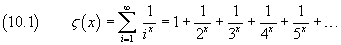If x=1, the (10.1) is the harmonic series, which don't converge. So for x=1 the function has a singularity.

The values of ζ(n), when n is an even natural number, can be exactly deduced. The method, found by L. Euler, may be the following.

• Starting from the MacLaurin series expansion of the sine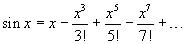when x≠0, one can get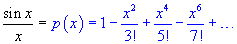p(x) has the same zeroes of sin x, excluding 0.

• In general, a polynomial P(x), with non-null zeroes xk and such that P(0)=1, may be factorized as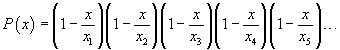Therefore p(x) may be written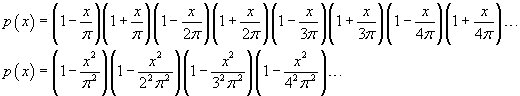• To fast the calculation, let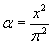; we get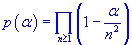The MacLaurin series expansion of p(α) is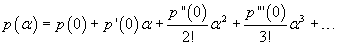• The first derivative of p(α) may be calculated using the logarithmic derivative method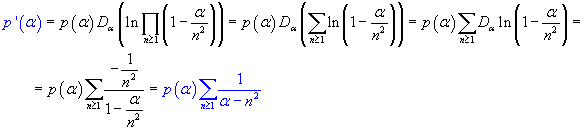For α=0 we get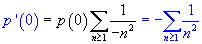p'(0)α must coincide with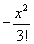, therefore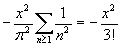Finally we get the value of ζ(2)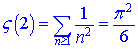• From p'(α) we can have p''(α)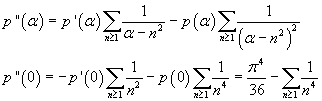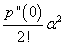must coincide with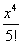, so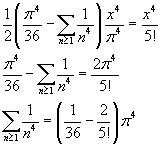From that we get the value of ζ(4)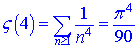• From p''(α) we can get p'''(α)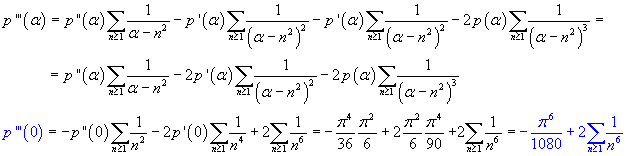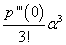must coincide with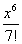, therefore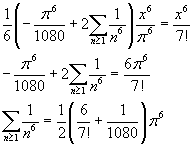Finally, the value of ζ(6) is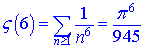These values of ζ are strictly correlated with the Bernoulli's numbers; they are particular cases of a general relation true for all the natural even numbers: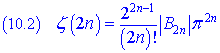From this relation we have also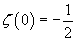The extension of the domain of ζ to the real and complex number shows that also the integer negative arguments generate values related to the Bernoulli's numbers. It can be demonstrated that, for natural numbers n≥2,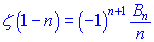that is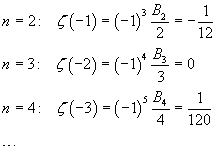The Bernoulli's numbers with odd index ≥ 3 are all 0, so the values of ζ for negative even integer values are all 0.

For arguments other than 1, the values of ζ can be numerically approximated (P. Borwein in "An Efficient Algorithm for the Riemann Zeta Function").

The following JavaScript application allows you to approximate the values of Z for real arguments. If your browser does not allow the `iframe` tag, you can open the source page.